﻿ Optical Transitions in Quantum Dots

Optical Transitions in Quantum Dots

A. Benahmed, A. Aissat, M. A. BenammarOPEN ACCESSPEER-REVIEWED

Optical Transitions in Quantum Dots

A. Benahmed1,, A. Aissat1, 2, M. A. Benammar1

1Faculty of the Sciences, FUNDAPL Laboratory, University of Blida.1, Algeria

2Faculty of the technology, LATSI Laboratory, University of Blida.1, Algeria

Abstract

The analysis of the electronic states of a quantum dot of InAs grown on a GaAs substrate has been studied for different geometries. We did the calculation with each type of geometry we based on the Schrödinger equation for stationary particle and we used Comsol for calculations. We calculated energy values as a function of each of the parameters: length, width and thickness of the wetting layer where other parameters are held constant.

At a glance: Figures

123
Prev Next

• Benahmed, A., A. Aissat, and M. A. Benammar. "Optical Transitions in Quantum Dots." International Journal of Physics 2.4 (2014): 109-111.
• Benahmed, A. , Aissat, A. , & Benammar, M. A. (2014). Optical Transitions in Quantum Dots. International Journal of Physics, 2(4), 109-111.
• Benahmed, A., A. Aissat, and M. A. Benammar. "Optical Transitions in Quantum Dots." International Journal of Physics 2, no. 4 (2014): 109-111.

 Import into BibTeX Import into EndNote Import into RefMan Import into RefWorks

1. Introduction

There are several ways to make quantum dots among these techniques include the method known as Stranski-Krastanov, which is the one used for the growth of quantum dots. The necessary condition for the achievement of a good course heterostructure is that the two materials have the same hand of a crystalline structure and other parameters of neighboring mesh . An important point is that this growth process results in the formation of a two-dimensional layer of InAs based uppermost islets called wetting layer, and acting as a reservoir of electrons scattered over a continuum of power levels [2, 3, 4]. InAs nanostructures may pose a potential for electronic confinement in combination with many semiconductor III-V as GaAs. In these nanostructures, the charge carriers are then sufficiently contained to allow the quantification of the energy levels of the system.  The objective of this work is to grow hetero structure from two semiconductor materials (InAs / GaAs). The gap in the InAs / GaAs system is low enough so that the corresponding optical transitions are in the visible or near infrared .We make calculations by "Comsol" with each type of geometry (rectangular, spherical and conical) at the same height and the same radius. To get a better idea of how energy changes indicates that we vary one of the parameters of the function (3). The energy level values obtained are listed in the Table 2.

2. Method

We detail the theoretical model that we used to determine the wave functions and energy Eigen states of electrons and holes through the resolution of the Schrödinger equation [2, 3].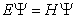(1)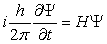(2)

The equation can be simplified to a stationary Schrödinger equation: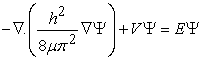(3)

The parameters of the equation are:

•  h (≈ 6,626.10-34 J.s) is Planck's constant.

•  μ is the reduced mass.

•  V is the potential energy.

•  E is the valueofenergy.

•  Ψ is the quantum mechanical wave function.

Where the periodicity condition implies that I the principal quantum number must be an integer. It remains to solve the equation 3, it is an instance of a PDE on the form factor, we will rewrite as: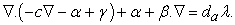• da is a damping coefficient or a mass coefficient.

• c is the diffusion coefficient.

• α is the conservative flux convection coefficient.

• β is the convection coefficient.

• a is the absorption coefficient.

• γ is the conservative flux source term.

Where the non-zero coefficients are: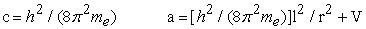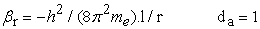And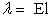.

We can model the overall structure in 2D as shown in Figure 1 below:

Whenever we will vary the values of the “wet “is the wetting layer thickness, “r” is the radius and “z “is the height of the QD.

Then, we present the results obtained from the numerical estimation used while interpreting these results in the modification of some parameters such as the radius, quantum dot height and thickness of the wetting layer. Indeed, the solutions of equation (3) where l = 0, which are transition energies depicted in Figure 6, Figure 7 and Figure 8. The expression of the transition energy is written as follows: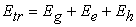(4)

Where Ee is the quantization energy of the electrons, Eh is the quantization energy of the holes and Eg is the energy gap.

Table 1. All parameters used in the calculation

To resolve this problem, we use the form PDE interface coefficient. The model solves for an eigenvalue / eigenvector. Electronvolt is used as an energy and nanometer length units of the geometry unit.

3. Result and Discuss

3.1. The electronic states of a Quantum Dot in as with Each Type of Geometry

The first step we choose is the realization of three different geometric structures shown in Figure 3, Figure 4 and Figure 5.We make calculations with Comsol each type of geometry with the same dimensions (height, radius, and thickness of the layer of wetting). The energy levels are listed in Table 1, and we see that the highest energy states are obtained for the conical quantum dot; we find that the energy is inversely proportional to the size of quantum dot.

Table 2. The energy levels for each geometry in the ground state E0 (eV)

3.2. Changes in the Parameters of the Quantum Dot

Among the three geometries, we have chosen the conical structure and we have made changes on the radius of the quantum dot "r" between (50A° and 250A°), the thickness and layer wettingand we obtained the results shown in the Figure 6, Figure 7 and Figure 8 respectively which correspond to energy levels of optical transition. The analysis of these figures shows that the transition energy is strongly dependent on the size of the quantum dot. Indeed, these energies are inversely proportional to the dimensions of the dot and to the thickness of the wetting layer.

Figure 8. The energy values for Changes in the witting layer thickness

There is an offset of the energy of transitions to higher energies because of the change in the confinement energy hen reduces the size of the structures [7, 8].

4. Conclusions

We have presented a review on the midinfrared properties of InAs/GaAs semiconductor self –assembled quantum dots. Our results obtained by studying a single quantum dot, were we use Comsol software and by simulation of elliptic, conical and rectangular nano-crystals InAs shows that the energy associated with the ground level depends on the shape and volume of quantum dots.

Acknowledgement

Our results obtained by studying a single quantum dot, were we use Comsol software and by simulation of elliptic, conical and rectangular nano-crystals InAs shows that the energy associated with the ground level depends on the shape and volume of quantum dots. We are hoping that this work will be helpful for experimentalists working on this material.

References

  H. Mathieu :« Physique des Semiconducteurs et des Composants Electronique», 6eme édition, pp (616-62 Paris, 2009).In article  COMSOL Multiphysics Model Library copyright 1998-2008 by COMSOL AB.In article  RF Module User’s Guide. 1998-2012 COMSOL-Protected by U.S. Patents 7, 519, 518; 7, 596, 474; and 7, 623, 991. Patents pending.In article  R. Chau, B. Doyle, S. Datta, J. Kavalieros and K. Zhang, Integrated nanoelectronics for the future, Nature Materials 6 (2007) 810.In article CrossRef  A. Abdelali: «Rôle du rayonnement dans l’élaboration et l’étude nanoagrégats semi-conducteur de ZnS », mémoire de magister, université de Mentouri Constantine.In article  P. M. Petroff, K. H. Schmidt, G. Medeiros-Ribeiro, A et J. Kotthaus, Size quantization and zero dimensional e in self assembled semiconductor quantum dots,Jpn. Jl. Appl Phys. 36, Part 1, 4068 (1997).In article CrossRef  C. Charles: «Propriétés électroniques, optiques et dynamiques de boites quantiques auto-organisées et couplées sur substrat InP », thèse doctorat, Institut National des Sciences Appliquées de Rennes, 27 juin 2006.In article  U. Banin, Y. Cao, D. Katz et O. Millo, Identification of atomic-like electronic states inindium arsenide nanocrystal quantum dots, Nature 400, 542 (1999).In article CrossRef